# Table 1 Multiplication and division in苏教版 Textbook (Grade 2 – Grade 4)

Grade-Semester Chapter Topic Content Example problem
Horizontal notation Vertical notation
2-1 1 Multiplication Meaning of multiplication; symbol (×) 4×2
2 Multiplication Multiplication tables from 1 to 6 (multiplier ≤6 and multiplicand ≤9) 1×1 6×9
4 Division Meaning of division; symbol (÷) 6÷2
5 Division Division problems with divisors from 1 to 6 (dividend ≤9 times divisor) 10÷2 36÷6
8 Multiplication and division Multiplication tables from 7 to 9 (multiplier and multiplicand ≤9) and related division problems 6×7
9×9
42÷7
81÷9

Vertical notation of multiplication and division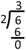2-2 1 Division Division with remainder; 1- or 2-digit divided by 1-digit 7÷3 17÷5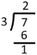8 Multiplication 2-digit multiplied by 1-digit 20×3
14×2 48×2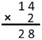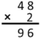3-1 1 Division 2-digit divided by 1-digit 40÷2
52÷2 62÷3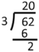7 Multiplication 3-digit multiplied by 1-digit 400×2
120×4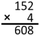3-2 1 Division 3-digit divided by 1-digit 600÷3
306÷34 Multiplication 2-digit multiplied by 2-digit 12×10
25×30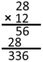4-1 1 Division 2- or 3-digit divided by 2-digit 60÷20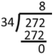4-2 1 Multiplication 3-digit multiplied by 2-digit